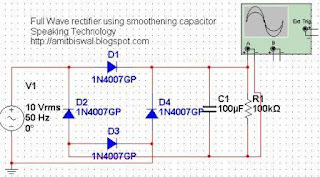Full wave bridge rectifier circuit [Multisim Simulation].

In one of my previous posts we studied about the half wave rectifier and simulated it using the National Instrument’s Multisim Simulation software. In this post, I would like to continue with the rectifier circuit, by explaining the full wave rectifier.

Basically a rectifier is usually used to rectify the pulses or waveforms of AC to DC. Another name of Full wave rectifier is Bridge rectifier as is mentioned in the title of this post.

This circuit consists of a set of 4 diodes connected in a bridge like structure. At any instant of time, only 2 diodes will be functional and other 2 will remain idle. In the positive half of the cycle, 2 diodes which are in forward bias mode will form the circuit and will conduct. In the negative half cycle, other 2 diodes which were earlier in reverse bias mode will be in forward bias mode and other 2 will be in reverse bias mode.

Coming to the simulation of this circuit, the circuit diagram drawn using the NI Multisim is shown below:full wave rectifier circuit diagram

You can see that 4 diodes are connected in a bridge kind of structure and the oscilloscope is connected across the resistor to observe the voltage waveforms across the resistor.

Here the components used are:

1. Sinusoidal voltage source: 10V, 50Hz
2. 4 diodes: 1N4007
3. Resistor: 100k ohms
4. Oscilloscope

When we run the simulation, only 2 diodes will conduct in each half cycle and will give rise to a voltage waveform which doesn’t contains any negative half of the original sinusoidal waveform. You can see the waveforms in the image given below:full wave rectifier output waveforms

After measuring using a probe, the rms voltage across the resistor is found to be 6.88V. So 10V signal is converted to 6.88V signal without having any negative cycle. Rest of the voltage i.e. (10-6.88) = 3.12V is the voltage drop across the diodes. Voltage drop across each diode is 1.56V.

But in practical dc application, we can’t use a DC with such a heavy ripples. So to reduce the ripples, we can make use of capacitors.

These ripple reducing capacitors are often termed as smoothening capacitor. In the circuit below, you can see that the electrolytic capacitor of 100uF has been connected in parallel to the resistor. This capacitor performs charging and discharging operation and converts the full wave rippled output to a smooth DC output across the resistor. The circuit with a smoothening capacitor is shown below:full wave rectifier using smoothening capacitor

The value of the capacitor shouldn’t be very small, otherwise it will not be effective in reducing the ripple to the required extent. Choosing an appropriate value can result in a very smooth DC output. In the image given below, you can see that 100uF capacitor works perfect with this circuit giving a perfectly smooth DC output. You can try simulating using a 100nF or other lesser valued capacitor, you will find the ripples which can be used to study the charging and discharging operations of the capacitor. The output waveforms are shown below:output waveform of full wave rectifier using smoothening capacitor

After measurement using a probe, it is found that the rms voltage across the resistor in this case is 8.26V.

1.Anonymous7/27/2012

fantastic post! keep working this cool stuff
My web site :: homes for sale in edinburg tx

2.Anonymous8/12/2012

This is a topic that's near to my heart... Many thanks! Exactly where are your contact details though?
Also visit my webpage - movies

3.Anonymous5/19/2013

preety nice. experimented with using different values of capacitors and saw the charging discharging thing.

1.keep experimenting, enjoy learning

4.Excellent explanation. Since exact calculation of current peak or true RMS of diode bridge is very hard due to high nonlinearity, some numerical simulation can be helpful.

http://www.cirvirlab.com/simulation/diode_bridge_online.php

5.6.Hi Amit,

Why the voltage drop across each diode too much. Can we calculate it?

7.I want to know if we connect a 1n4007 dide to bridge rectifier output , what will be the output voltage?

If you liked this blog then Join me at: